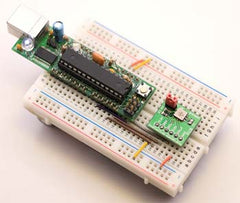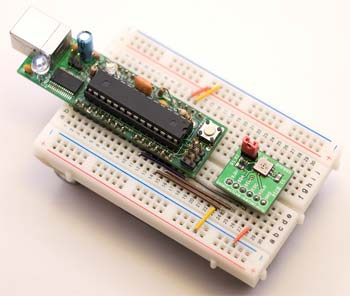# 🌦 Do-it-yourself electronic barometer

In this project, we will consider connecting the pressure sensor on the I2C interface to the Arduino controller and taking readings. Simply put, we will create an electronic barometer with our own hands.

As a pressure sensor, we will use the BMP085 from Bosch.
The BMP085 is a high-precision, ultra-low power, high-precision digital atmospheric pressure sensor. Also, the sensor allows you to measure temperature. Connection interface: I2C. The sensor is used in GPS navigation, weather stations, instruments for determining the speed of ascent/descent, in measuring the power of the air flow, etc.The information provided by Bosch to work with this sensor is very scarce. Jeelabs website helped to deal with BMP085

HardwareTo Arduino, the BMP085 sensor is connected in the same way as other I2C devices: connect VCC to VCC, GND to GND, SCL to analog output 5, and SDA to analog output 4. Put pull-up resistors (from 1k to 20k, for example, 4.7kOhm between SDA, SCL and VCC (they are already on my board).

Sensor supply voltage: 1.8-3.6 V. Also, the sensor contains an EOC output, which signals the end of the measurement and data processing process. If EOC = 1, then processing is completed, if EOC = 0, then in the process. The EOC is connected to the Arduino 2 analog output, but not used.

Software
To our delight, the code from Jeenode contains all the necessary functionality, the only thing I added is the ability to use all oversampling modes. The BMP085 has the ability to set 4 oversampling modes, each of which spends more time and energy than the previous mode, but at the same time improves the measurement accuracy.

So, the program. First, we need to make it possible to read all 16-bit values ​​from the sensor registers:

```int read_int_register(unsigned char r)
{
unsigned char msb, lsb;
Wire.send(r);
Wire.endTransmission();

while(!Wire.available()) {
}
while(!Wire.available()) {
}
return (((int)msb<<8) | ((int)lsb));
}```

Next, we need a function that will write the value in the 8-bit register:

```char read_register(unsigned char r)
{
unsigned char v;
Wire.send(r);
Wire.endTransmission();

while(!Wire.available()) {
}
return v;
}```

Then, you need to define several global variables to read the calibration data from the EEPROM sensor:

int ac1; int ac2; int ac3; unsigned int ac4; unsigned int ac5; unsigned int ac6; int b1; int b2; int mb; int mc; int md;

Taking readings of all values with EEPROM can be more efficient than writing and reading readings from each register every time. So A good time gain is achieved.
The raw temperature (ut) and pressure (up) data can be read as 16 and 24-bit values:

```unsigned int bmp085_read_ut() {
write_register(0xf4,0x2e);
delay(5);
}
```
```long bmp085_read_up() {
write_register(0xf4,0x34+(oversampling_setting<<6));
delay(pressure_waittime[oversampling_setting]);

unsigned char msb, lsb, xlsb;
Wire.send(0xf6);
Wire.endTransmission();

while(!Wire.available()) {
}
while(!Wire.available()) {
}
while(!Wire.available()) {
}
return (((long)msb<<16) | ((long)lsb<<8) | ((long)xlsb)) >>(8-oversampling_setting);
}```

Алгоритм преобразования температуры и давления из raw-данных датчика в реальную температуру (градусы Цельсия) и давление (Паскаль) взят из даташита (плюс некоторые дополнения от Jeenodes):

```void bmp085_read_temperature_and_pressure(int* temperature, long* pressure) {
long x1, x2, x3, b3, b5, b6, p;
unsigned long b4, b7;

//расчет температуры
x1 = ((long)ut - ac6) * ac5 >> 15;
x2 = ((long) mc << 11) / (x1 + md);
b5 = x1 + x2;
*temperature = (b5 + 8) >> 4;

//расчет давления
b6 = b5 - 4000;
x1 = (b2 * (b6 * b6 >> 12)) >> 11;
x2 = ac2 * b6 >> 11;
x3 = x1 + x2;
b3 = (((int32_t) ac1 * 4 + x3)<> 2;
x1 = ac3 * b6 >> 13;
x2 = (b1 * (b6 * b6 >> 12)) >> 16;
x3 = ((x1 + x2) + 2) >> 2;
b4 = (ac4 * (uint32_t) (x3 + 32768)) >> 15;
b7 = ((uint32_t) up - b3) * (50000 >> oversampling_setting);
p = b7 < 0x80000000 ? (b7 * 2) / b4 : (b7 / b4) * 2;

x1 = (p >> 8) * (p >> 8);
x1 = (x1 * 3038) >> 16;
x2 = (-7357 * p) >> 16;
*pressure = p + ((x1 + x2 + 3791) >> 4);
}```

Temperature and pressure data are calculated at the same time (temperature values are used to calculate pressure).